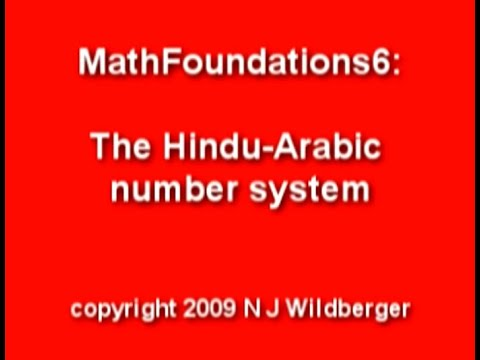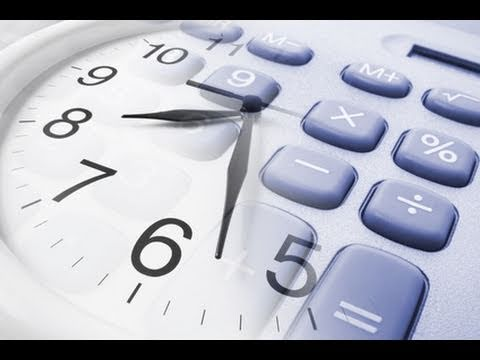## Hindu Arabic Numeration System

Subject: Compulsory Maths

#### Overview

It is the number system in which ten digits (i.e. 0, 1, 2, 3, 4, 5, 6, 7, 8 ,9 ) are used. Any whole number can be written using a combination of these ten digits. As it is based on the grouping of tens, it is also known as Decimal Number System or the Base Ten System. The value of each digit in a number depends on its place in the number.

### Hindu-Arabic Number

It is the number system in which ten digits (i.e. 0, 1, 2, 3, 4, 5, 6, 7, 8 ,9 ) are used. Any whole number can be written using a combination of these ten digits. As it is based on the grouping of tens, it is also known as Decimal Number System or the Base Ten System. The value of each digit in a number depends on its place in the number. For example:
6 9 8 2

Thus, we can obtain the place value of any digit in a number by multiplying the digit by its place. So,the above number can also be expressed in expanded form as:
6982 = 6× 1000 + 9× 100 + 8× 10 + 2× 1
= 6× 103 + 9× 102 + 8× 101 + 2× 100 [$\because$ 100 = 1]#### Periods and places in Nepali and in International System

Nepali System
The table below shows the periods and places of number in Nepali system:

 Periods Kharabs Arabs Crores Lakhs Thousands Units Places Ten Kharabs Kharabs Ten Arabs Arabs Ten Crores Crores Ten Lakhs Lakhs Ten Thousand Thousand Hundred Tens Once Power of 10 1012 1011 1010 109 108 107 106 105 104 103 102 101 100 Number 6 9 2 4 3 1 7 2 8 0 1 6

From the above table, the number name of 692431728016 is:
Six kharab ninety-two arab forty-three crores seventeen lakh twenty-eight thousand sixteen.

International System

 Periods Bllions Millions Thousands Units Places HundredBillions TenBillions Billions HundredMillions TenMillions Millions HundredThousands TenThousand Thousands Hundreds Tens Once Power of 10 1011 1010 109 108 107 106 105 104 103 102 101 100 Number 6 9 2 4 3 1 7 2 8 0 1 6

In International system, the number name of 692431728016:
Six hundred ninety-two billion four hundred thirty-one million seven hundred twenty-eight thousand sixteen.

#### Use of Commas

1. Use of commas in Nepali system
At first, units period is separated, then the numbers of places of thousand, lakhs, crores etc are separated. For examples, 692431728016 is written as Six kharab ninety-two arab forty-three crores seventeen lakh twenty-eight thousand sixteen.
2. Use of commas International systemsource: www.flexiprep.comFig: International Commas System At first, units period is separated, then the numbers of places of thousands, millions and billions are separated. For example,692431728016 is written as Six hundred ninety-two billion four hundred thirty-one million seven hundred twenty-eight thousand sixteen.
##### Things to remember
• It is the number system in which ten digits (i.e. 0, 1, 2, 3, 4, 5, 6, 7, 8 ,9 ) are used. Any whole number can be written using a combination of these ten digits. As it is based on the grouping of tens, it is also known as Decimal Number System or the Base Ten System.

• It includes every relationship which established among the people.
• There can be more than one community in a society. Community smaller than society.
• It is a network of social relationships which cannot see or touched.
• common interests and common objectives are not necessary for society.
##### Videos for Hindu Arabic Numeration System##### MathFoundations6: The Hindu-Arabic number system##### Numeral System, Hindu-Arabic System

Solution:

In Nepali System,
48,59,13,082 = Forty-eight crores fifty-nine lakhs thirteen thousands and eighty-two.

In International System,
485,913,082 = Four hundred eighty-five million nine hundred thirteen thousand and eighty-two.

Solution:

In Nepali system,
fifteen crores twenty lakh kilometer = 15,20,00,000

In International system,
fifteen crore twenty lakh kilometer = 152, 000, 000
One hundred fifty two million.

Solution:

There are 10 lakhs in 1 million

Solution;

In Nepali system,
268795438 =  2 × 108 + 6 × 107 + 8 × 106 + 7 × 105 + 9 × 104 + 5 × 103 + 4 × 102 + 3  × 10 1 + 8 × 100

Solution:

In Nepali system,
7,23,60,95,841 = Seven arab twenty-three crore sixty lakh ninety-five thousand eight hundred and forty-one.

In International system,
7,236,095,841 = Seven billion two hundred thirty-six million ninety-five thousand eight hundred and forty-one.

Solution:

In Nepali system,
9,05,73,02,18,645 = Nine kharab five arab seventy-three crore two lakh eighteen thousand six hundred and forty five.

In International system,
905,730,218,645 = Nine hundred five billion seven hundred thirty million two hundred eighteen thousand six hundred and forty-five.

Solution:

8 × 1011 + 4 × 108 + 9 × 10+ 7 × 103 + 6 × 102 + 2 × 101 + 3 × 100

Solution:

It is Ten arab of ten billion in Nepali system.